# HashMap 源码详细分析（JDK1.8）

+关注继续查看

## 二、原理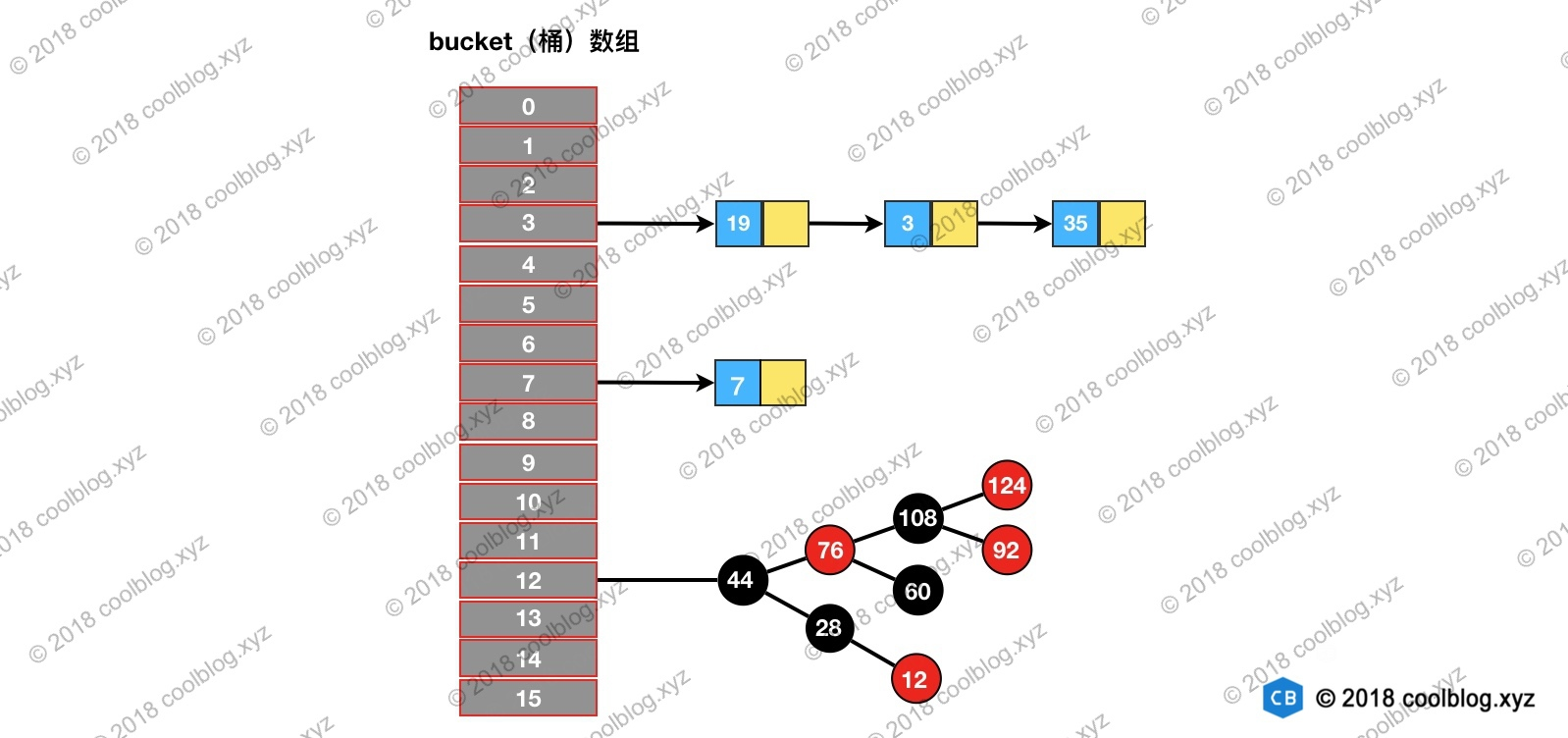1. 定位元素35所处桶的位置，index = 35 % 16 = 3
2. 3号桶所指向的链表中继续查找，发现35在链表中。

## 三、源码分析

### 3.1 构造方法

#### 3.1.1 构造方法分析

HashMap 的构造方法不多，只有四个。HashMap 构造方法做的事情比较简单，一般都是初始化一些重要变量，比如 loadFactor 和 threshold。而底层的数据结构则是延迟到插入键值对时再进行初始化。HashMap 相关构造方法如下：

/** 构造方法 1 */
public HashMap() {
}

/** 构造方法 2 */
public HashMap(int initialCapacity) {
}

/** 构造方法 3 */
public HashMap(int initialCapacity, float loadFactor) {
if (initialCapacity < 0)
throw new IllegalArgumentException("Illegal initial capacity: " +
initialCapacity);
if (initialCapacity > MAXIMUM_CAPACITY)
initialCapacity = MAXIMUM_CAPACITY;
throw new IllegalArgumentException("Illegal load factor: " +
this.threshold = tableSizeFor(initialCapacity);
}

/** 构造方法 4 */
public HashMap(Map<? extends K, ? extends V> m) {
putMapEntries(m, false);
}

#### 3.1.2 初始容量、负载因子、阈值

initialCapacity HashMap 初始容量
threshold 当前 HashMap 所能容纳键值对数量的最大值，超过这个值，则需扩容

/** The default initial capacity - MUST be a power of two. */
static final int DEFAULT_INITIAL_CAPACITY = 1 << 4;

/** The load factor used when none specified in constructor. */
static final float DEFAULT_LOAD_FACTOR = 0.75f;

/** The next size value at which to resize (capacity * load factor). */
int threshold;

/**
* Returns a power of two size for the given target capacity.
*/
static final int tableSizeFor(int cap) {
int n = cap - 1;
n |= n >>> 1;
n |= n >>> 2;
n |= n >>> 4;
n |= n >>> 8;
n |= n >>> 16;
return (n < 0) ? 1 : (n >= MAXIMUM_CAPACITY) ? MAXIMUM_CAPACITY : n + 1;
}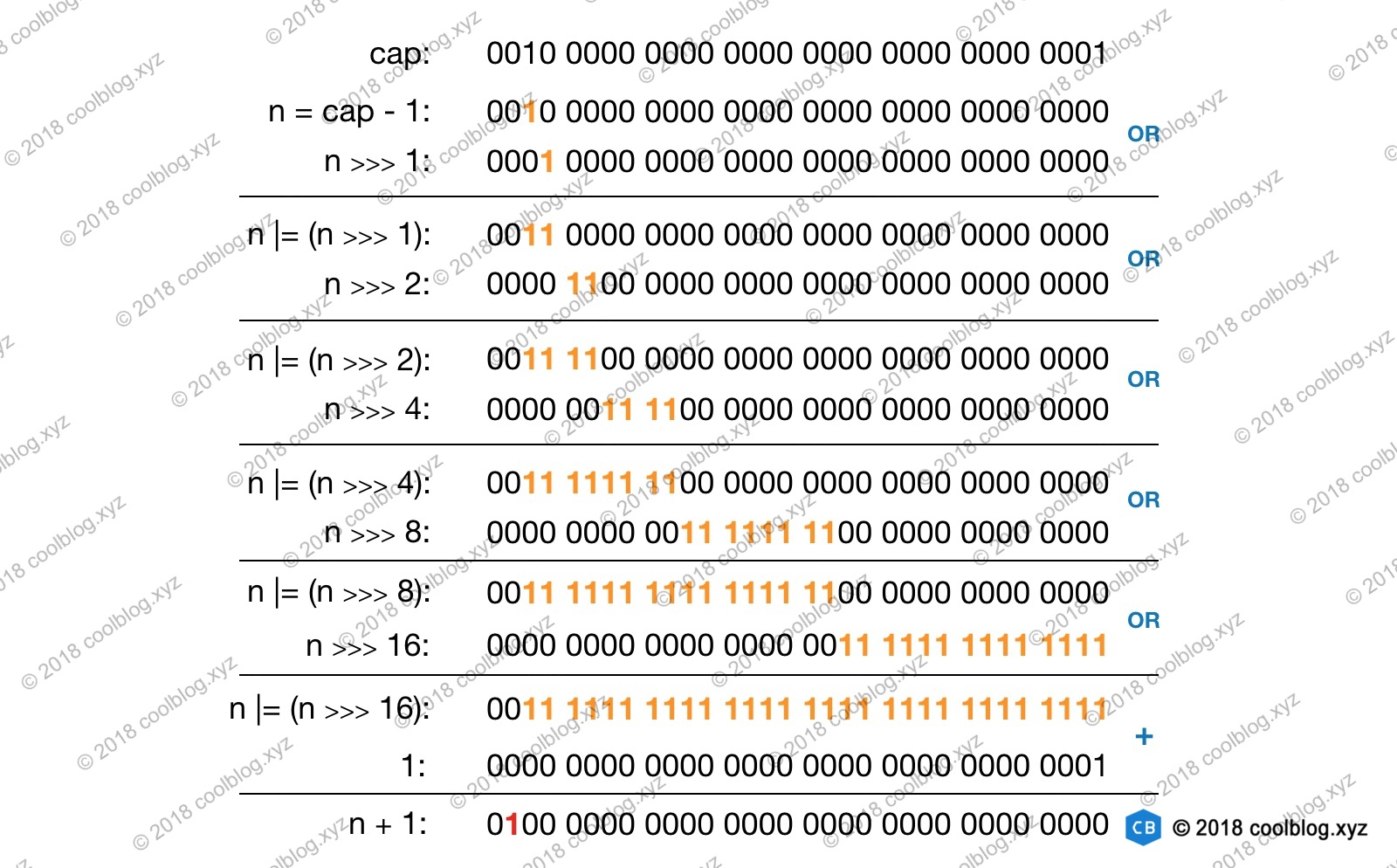### 3.2 查找

HashMap 的查找操作比较简单，查找步骤与原理篇介绍一致，即先定位键值对所在的桶的位置，然后再对链表或红黑树进行查找。通过这两步即可完成查找，该操作相关代码如下：

public V get(Object key) {
Node<K,V> e;
return (e = getNode(hash(key), key)) == null ? null : e.value;
}

final Node<K,V> getNode(int hash, Object key) {
Node<K,V>[] tab; Node<K,V> first, e; int n; K k;
// 1. 定位键值对所在桶的位置
if ((tab = table) != null && (n = tab.length) > 0 &&
(first = tab[(n - 1) & hash]) != null) {
if (first.hash == hash && // always check first node
((k = first.key) == key || (key != null && key.equals(k))))
return first;
if ((e = first.next) != null) {
// 2. 如果 first 是 TreeNode 类型，则调用黑红树查找方法
if (first instanceof TreeNode)
return ((TreeNode<K,V>)first).getTreeNode(hash, key);

// 2. 对链表进行查找
do {
if (e.hash == hash &&
((k = e.key) == key || (key != null && key.equals(k))))
return e;
} while ((e = e.next) != null);
}
}
return null;
}

// index = (n - 1) & hash
first = tab[(n - 1) & hash]/**
* 计算键的 hash 值
*/
static final int hash(Object key) {
int h;
return (key == null) ? 0 : (h = key.hashCode()) ^ (h >>> 16);
}### 3.3 遍历

for(Object key : map.keySet()) {
// do something
}

for(HashMap.Entry entry : map.entrySet()) {
// do something
}

Set keys = map.keySet();
Iterator ite = keys.iterator();
while (ite.hasNext()) {
Object key = ite.next();
// do something
}

public Set<K> keySet() {
Set<K> ks = keySet;
if (ks == null) {
ks = new KeySet();
keySet = ks;
}
return ks;
}

/**
* 键集合
*/
final class KeySet extends AbstractSet<K> {
public final int size()                 { return size; }
public final void clear()               { HashMap.this.clear(); }
public final Iterator<K> iterator()     { return new KeyIterator(); }
public final boolean contains(Object o) { return containsKey(o); }
public final boolean remove(Object key) {
return removeNode(hash(key), key, null, false, true) != null;
}
// 省略部分代码
}

/**
* 键迭代器
*/
final class KeyIterator extends HashIterator
implements Iterator<K> {
public final K next() { return nextNode().key; }
}

abstract class HashIterator {
Node<K,V> next;        // next entry to return
Node<K,V> current;     // current entry
int expectedModCount;  // for fast-fail
int index;             // current slot

HashIterator() {
expectedModCount = modCount;
Node<K,V>[] t = table;
current = next = null;
index = 0;
if (t != null && size > 0) { // advance to first entry
// 寻找第一个包含链表节点引用的桶
do {} while (index < t.length && (next = t[index++]) == null);
}
}

public final boolean hasNext() {
return next != null;
}

final Node<K,V> nextNode() {
Node<K,V>[] t;
Node<K,V> e = next;
if (modCount != expectedModCount)
throw new ConcurrentModificationException();
if (e == null)
throw new NoSuchElementException();
if ((next = (current = e).next) == null && (t = table) != null) {
// 寻找下一个包含链表节点引用的桶
do {} while (index < t.length && (next = t[index++]) == null);
}
return e;
}
//省略部分代码
}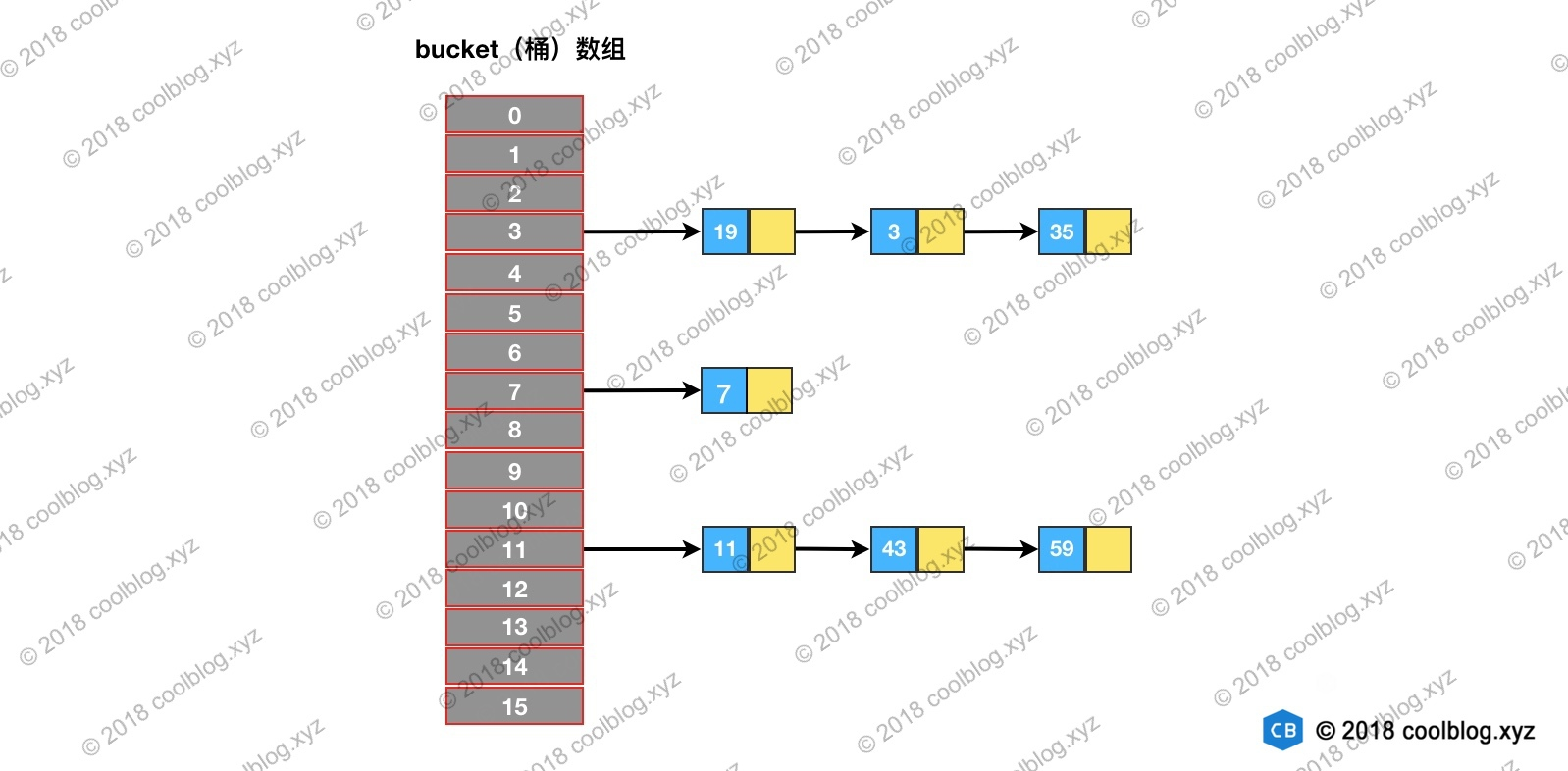HashIterator 在初始化时，会先遍历桶数组，找到包含链表节点引用的桶，对应图中就是3号桶。随后由 nextNode 方法遍历该桶所指向的链表。遍历完3号桶后，nextNode 方法继续寻找下一个不为空的桶，对应图中的7号桶。之后流程和上面类似，直至遍历完最后一个桶。以上就是 HashIterator 的核心逻辑的流程，对应下图：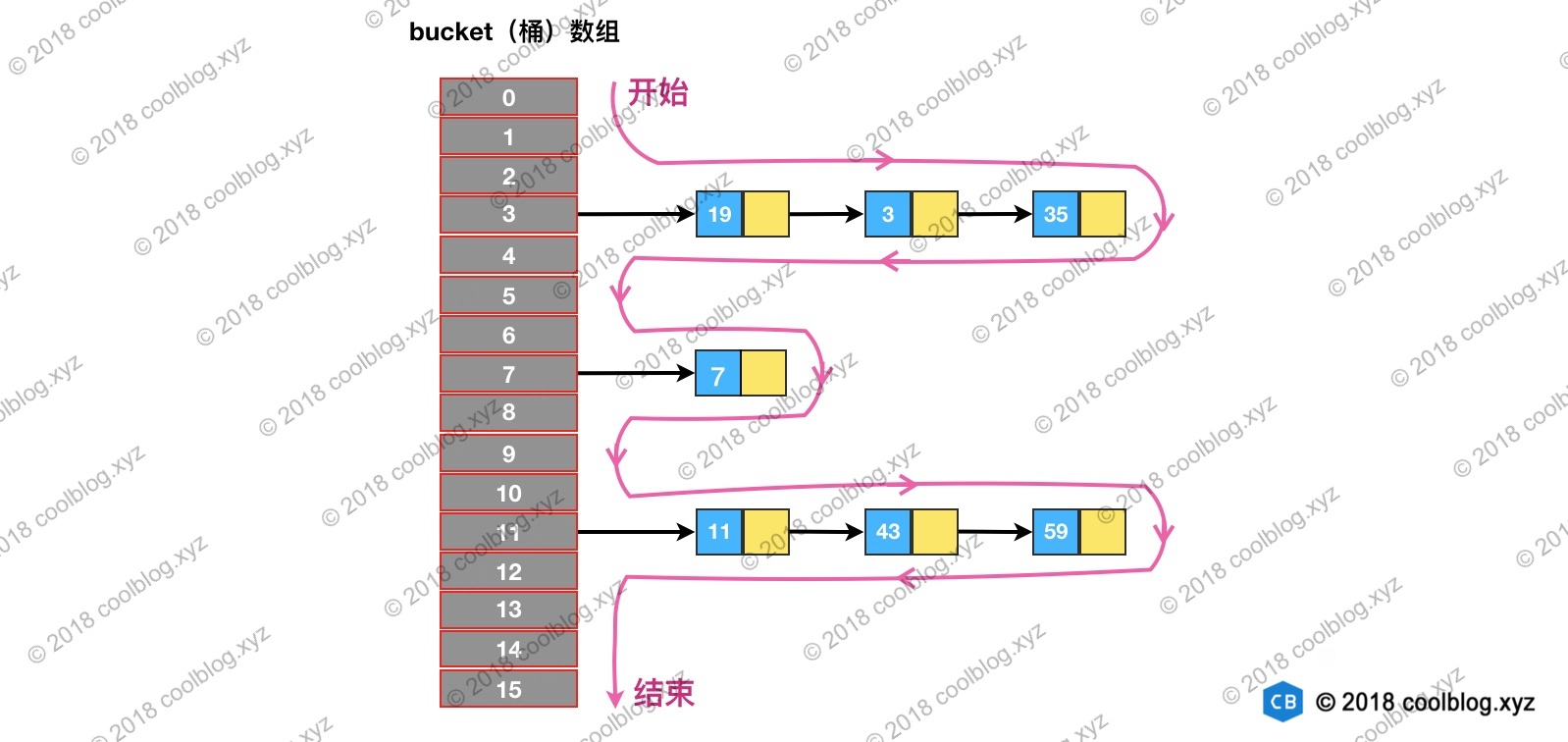/**
* 应在 JDK 1.8 下测试，其他环境下不保证结果和上面一致
*/
public class HashMapTest {

@Test
public void testTraversal() {
HashMap<Integer, String> map = new HashMap(16);
map.put(7, "");
map.put(11, "");
map.put(43, "");
map.put(59, "");
map.put(19, "");
map.put(3, "");
map.put(35, "");

System.out.println("遍历结果：");
for (Integer key : map.keySet()) {
System.out.print(key + " -> ");
}
}
}

### 3.4 插入

#### 3.4.1 插入逻辑分析

public V put(K key, V value) {
return putVal(hash(key), key, value, false, true);
}

final V putVal(int hash, K key, V value, boolean onlyIfAbsent,
boolean evict) {
Node<K,V>[] tab; Node<K,V> p; int n, i;
// 初始化桶数组 table，table 被延迟到插入新数据时再进行初始化
if ((tab = table) == null || (n = tab.length) == 0)
n = (tab = resize()).length;
// 如果桶中不包含键值对节点引用，则将新键值对节点的引用存入桶中即可
if ((p = tab[i = (n - 1) & hash]) == null)
tab[i] = newNode(hash, key, value, null);
else {
Node<K,V> e; K k;
// 如果键的值以及节点 hash 等于链表中的第一个键值对节点时，则将 e 指向该键值对
if (p.hash == hash &&
((k = p.key) == key || (key != null && key.equals(k))))
e = p;

// 如果桶中的引用类型为 TreeNode，则调用红黑树的插入方法
else if (p instanceof TreeNode)
e = ((TreeNode<K,V>)p).putTreeVal(this, tab, hash, key, value);
else {
// 对链表进行遍历，并统计链表长度
for (int binCount = 0; ; ++binCount) {
// 链表中不包含要插入的键值对节点时，则将该节点接在链表的最后
if ((e = p.next) == null) {
p.next = newNode(hash, key, value, null);
// 如果链表长度大于或等于树化阈值，则进行树化操作
if (binCount >= TREEIFY_THRESHOLD - 1) // -1 for 1st
treeifyBin(tab, hash);
break;
}

// 条件为 true，表示当前链表包含要插入的键值对，终止遍历
if (e.hash == hash &&
((k = e.key) == key || (key != null && key.equals(k))))
break;
p = e;
}
}

// 判断要插入的键值对是否存在 HashMap 中
if (e != null) { // existing mapping for key
V oldValue = e.value;
// onlyIfAbsent 表示是否仅在 oldValue 为 null 的情况下更新键值对的值
if (!onlyIfAbsent || oldValue == null)
e.value = value;
afterNodeAccess(e);
return oldValue;
}
}
++modCount;
// 键值对数量超过阈值时，则进行扩容
if (++size > threshold)
resize();
afterNodeInsertion(evict);
return null;
}

1. 当桶数组 table 为空时，通过扩容的方式初始化 table
2. 查找要插入的键值对是否已经存在，存在的话根据条件判断是否用新值替换旧值
3. 如果不存在，则将键值对链入链表中，并根据链表长度决定是否将链表转为红黑树
4. 判断键值对数量是否大于阈值，大于的话则进行扩容操作

#### 3.4.2 扩容机制

HashMap 的扩容机制与其他变长集合的套路不太一样，HashMap 按当前桶数组长度的2倍进行扩容，阈值也变为原来的2倍（如果计算过程中，阈值溢出归零，则按阈值公式重新计算）。扩容之后，要重新计算键值对的位置，并把它们移动到合适的位置上去。以上就是 HashMap 的扩容大致过程，接下来我们来看看具体的实现：

final Node<K,V>[] resize() {
Node<K,V>[] oldTab = table;
int oldCap = (oldTab == null) ? 0 : oldTab.length;
int oldThr = threshold;
int newCap, newThr = 0;
// 如果 table 不为空，表明已经初始化过了
if (oldCap > 0) {
// 当 table 容量超过容量最大值，则不再扩容
if (oldCap >= MAXIMUM_CAPACITY) {
threshold = Integer.MAX_VALUE;
return oldTab;
}
// 按旧容量和阈值的2倍计算新容量和阈值的大小
else if ((newCap = oldCap << 1) < MAXIMUM_CAPACITY &&
oldCap >= DEFAULT_INITIAL_CAPACITY)
newThr = oldThr << 1; // double threshold
} else if (oldThr > 0) // initial capacity was placed in threshold
/*
* 初始化时，将 threshold 的值赋值给 newCap，
* HashMap 使用 threshold 变量暂时保存 initialCapacity 参数的值
*/
newCap = oldThr;
else {               // zero initial threshold signifies using defaults
/*
* 调用无参构造方法时，桶数组容量为默认容量，
* 阈值为默认容量与默认负载因子乘积
*/
newCap = DEFAULT_INITIAL_CAPACITY;
}

// newThr 为 0 时，按阈值计算公式进行计算
if (newThr == 0) {
float ft = (float)newCap * loadFactor;
newThr = (newCap < MAXIMUM_CAPACITY && ft < (float)MAXIMUM_CAPACITY ?
(int)ft : Integer.MAX_VALUE);
}
threshold = newThr;
// 创建新的桶数组，桶数组的初始化也是在这里完成的
Node<K,V>[] newTab = (Node<K,V>[])new Node[newCap];
table = newTab;
if (oldTab != null) {
// 如果旧的桶数组不为空，则遍历桶数组，并将键值对映射到新的桶数组中
for (int j = 0; j < oldCap; ++j) {
Node<K,V> e;
if ((e = oldTab[j]) != null) {
oldTab[j] = null;
if (e.next == null)
newTab[e.hash & (newCap - 1)] = e;
else if (e instanceof TreeNode)
// 重新映射时，需要对红黑树进行拆分
((TreeNode<K,V>)e).split(this, newTab, j, oldCap);
else { // preserve order
Node<K,V> loHead = null, loTail = null;
Node<K,V> hiHead = null, hiTail = null;
Node<K,V> next;
// 遍历链表，并将链表节点按原顺序进行分组
do {
next = e.next;
if ((e.hash & oldCap) == 0) {
if (loTail == null)
else
loTail.next = e;
loTail = e;
}
else {
if (hiTail == null)
else
hiTail.next = e;
hiTail = e;
}
} while ((e = next) != null);
// 将分组后的链表映射到新桶中
if (loTail != null) {
loTail.next = null;
}
if (hiTail != null) {
hiTail.next = null;
}
}
}
}
}
return newTab;
}

1. 计算新桶数组的容量 newCap 和新阈值 newThr
2. 根据计算出的 newCap 创建新的桶数组，桶数组 table 也是在这里进行初始化的
3. 将键值对节点重新映射到新的桶数组里。如果节点是 TreeNode 类型，则需要拆分红黑树。如果是普通节点，则节点按原顺序进行分组。

// 第一个条件分支
if ( oldCap > 0) {
// 嵌套条件分支
if (oldCap >= MAXIMUM_CAPACITY) {...}
else if ((newCap = oldCap << 1) < MAXIMUM_CAPACITY &&
oldCap >= DEFAULT_INITIAL_CAPACITY) {...}
}
else if (oldThr > 0) {...}
else {...}

// 第二个条件分支
if (newThr == 0) {...}

oldCap > 0 桶数组 table 已经被初始化
oldThr > 0 threshold > 0，且桶数组未被初始化 调用 HashMap(int) 和 HashMap(int, float) 构造方法时会产生这种情况，此种情况下 newCap = oldThr，newThr 在第二个条件分支中算出
oldCap == 0 && oldThr == 0 桶数组未被初始化，且 threshold 为 0 调用 HashMap() 构造方法会产生这种情况。

oldCap >= 230 桶数组容量大于或等于最大桶容量 230 这种情况下不再扩容
newCap < 230 && oldCap > 16 新桶数组容量小于最大值，且旧桶数组容量大于 16 该种情况下新阈值 newThr = oldThr << 1，移位可能会导致溢出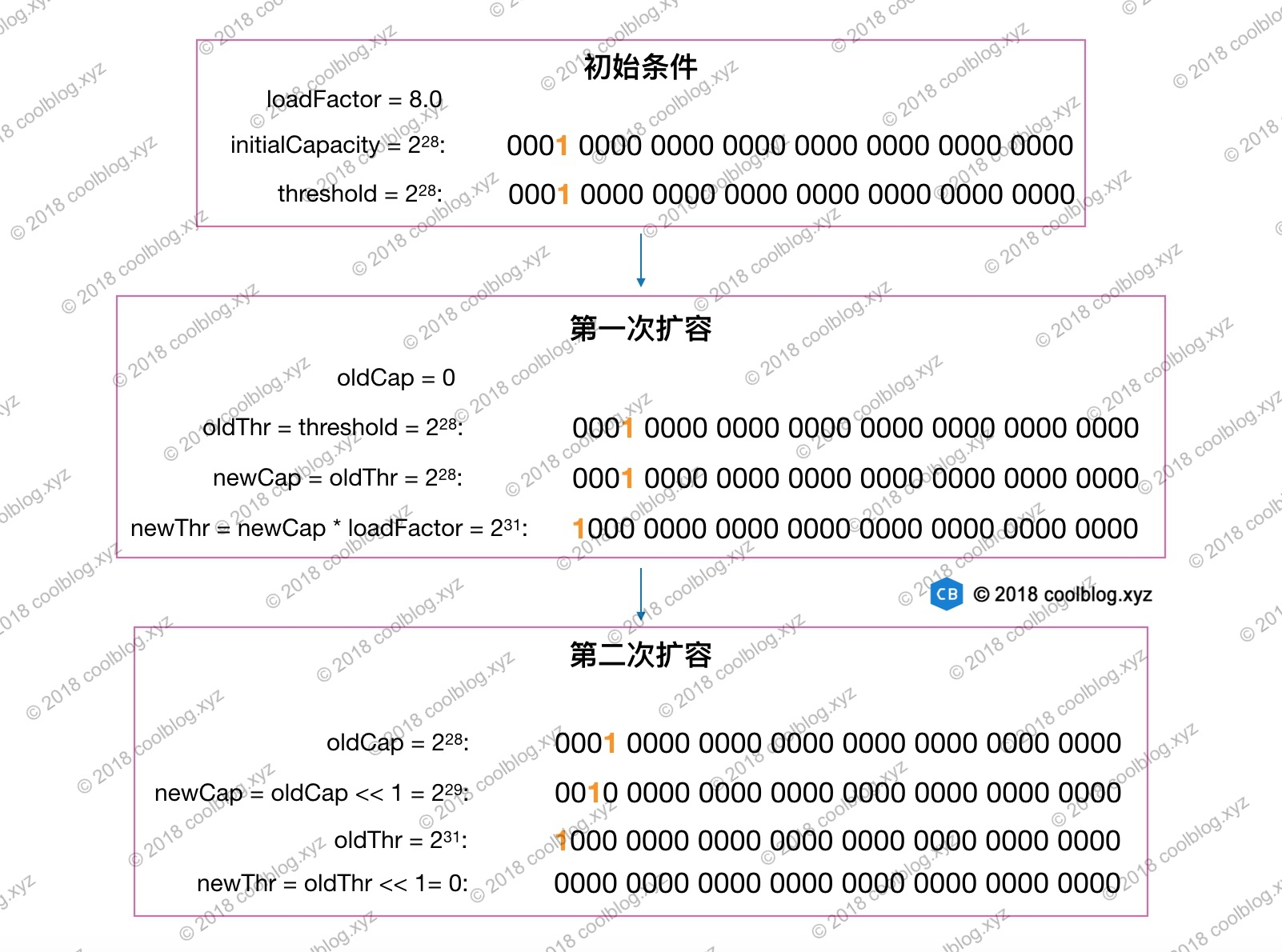newThr == 0 第一个条件分支未计算 newThr 或嵌套分支在计算过程中导致 newThr 溢出归零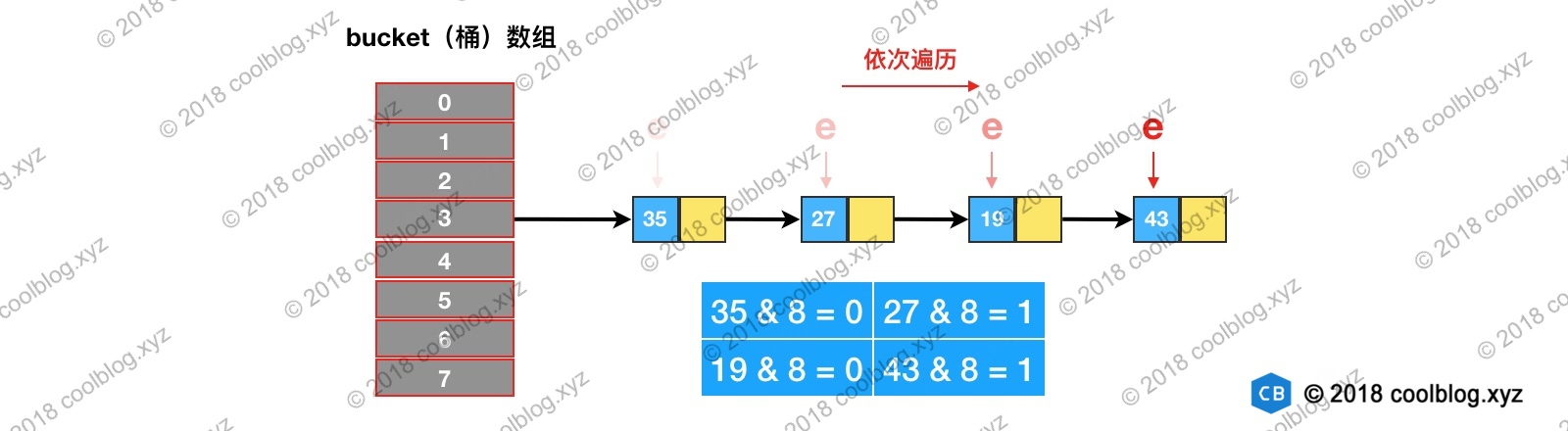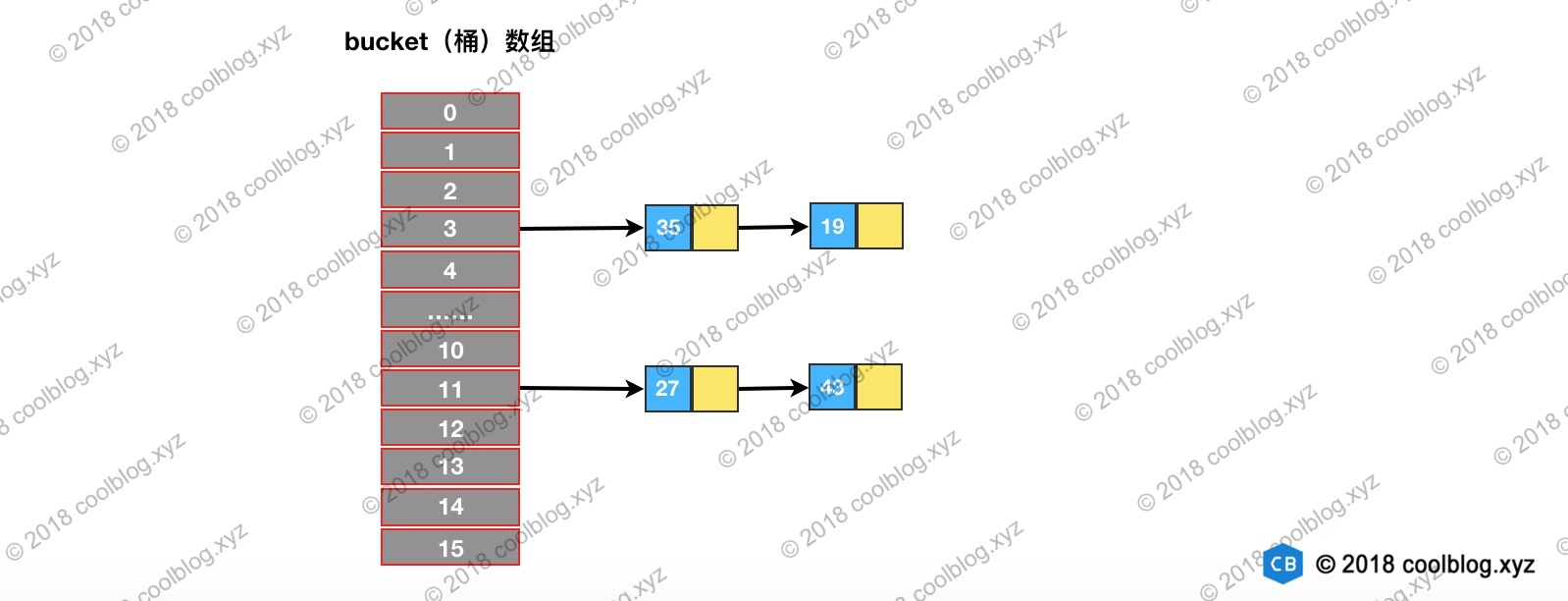#### 3.4.3 链表树化、红黑树链化与拆分

JDK 1.8 对 HashMap 实现进行了改进。最大的改进莫过于在引入了红黑树处理频繁的碰撞，代码复杂度也随之上升。比如，以前只需实现一套针对链表操作的方法即可。而引入红黑树后，需要另外实现红黑树相关的操作。红黑树是一种自平衡的二叉查找树，本身就比较复杂。本篇文章中并不打算对红黑树展开介绍，本文仅会介绍链表树化需要注意的地方。至于红黑树详细的介绍，如果大家有兴趣，可以参考我的另一篇文章 - 红黑树详细分析

static final int TREEIFY_THRESHOLD = 8;

/**
* 当桶数组容量小于该值时，优先进行扩容，而不是树化
*/
static final int MIN_TREEIFY_CAPACITY = 64;

static final class TreeNode<K,V> extends LinkedHashMap.Entry<K,V> {
TreeNode<K,V> parent;  // red-black tree links
TreeNode<K,V> left;
TreeNode<K,V> right;
TreeNode<K,V> prev;    // needed to unlink next upon deletion
boolean red;
TreeNode(int hash, K key, V val, Node<K,V> next) {
super(hash, key, val, next);
}
}

/**
* 将普通节点链表转换成树形节点链表
*/
final void treeifyBin(Node<K,V>[] tab, int hash) {
int n, index; Node<K,V> e;
// 桶数组容量小于 MIN_TREEIFY_CAPACITY，优先进行扩容而不是树化
if (tab == null || (n = tab.length) < MIN_TREEIFY_CAPACITY)
resize();
else if ((e = tab[index = (n - 1) & hash]) != null) {
TreeNode<K,V> hd = null, tl = null;
do {
// 将普通节点替换成树形节点
TreeNode<K,V> p = replacementTreeNode(e, null);
if (tl == null)
hd = p;
else {
p.prev = tl;
tl.next = p;
}
tl = p;
} while ((e = e.next) != null);  // 将普通链表转成由树形节点链表
if ((tab[index] = hd) != null)
// 将树形链表转换成红黑树
hd.treeify(tab);
}
}

TreeNode<K,V> replacementTreeNode(Node<K,V> p, Node<K,V> next) {
return new TreeNode<>(p.hash, p.key, p.value, next);
}

1. 链表长度大于等于 TREEIFY_THRESHOLD
2. 桶数组容量大于等于 MIN_TREEIFY_CAPACITYHashMap 在设计之初，并没有考虑到以后会引入红黑树进行优化。所以并没有像 TreeMap 那样，要求键类实现 comparable 接口或提供相应的比较器。但由于树化过程需要比较两个键对象的大小，在键类没有实现 comparable 接口的情况下，怎么比较键与键之间的大小了就成了一个棘手的问题。为了解决这个问题，HashMap 是做了三步处理，确保可以比较出两个键的大小，如下：

1. 比较键与键之间 hash 的大小，如果 hash 相同，继续往下比较
2. 检测键类是否实现了 Comparable 接口，如果实现调用 compareTo 方法进行比较
3. 如果仍未比较出大小，就需要进行仲裁了，仲裁方法为 tieBreakOrder（大家自己看源码吧）

tie break 是网球术语，可以理解为加时赛的意思，起这个名字还是挺有意思的。##### 红黑树拆分

// 红黑树转链表阈值
static final int UNTREEIFY_THRESHOLD = 6;

final void split(HashMap<K,V> map, Node<K,V>[] tab, int index, int bit) {
TreeNode<K,V> b = this;
// Relink into lo and hi lists, preserving order
TreeNode<K,V> loHead = null, loTail = null;
TreeNode<K,V> hiHead = null, hiTail = null;
int lc = 0, hc = 0;
/*
* 红黑树节点仍然保留了 next 引用，故仍可以按链表方式遍历红黑树。
* 下面的循环是对红黑树节点进行分组，与上面类似
*/
for (TreeNode<K,V> e = b, next; e != null; e = next) {
next = (TreeNode<K,V>)e.next;
e.next = null;
if ((e.hash & bit) == 0) {
if ((e.prev = loTail) == null)
else
loTail.next = e;
loTail = e;
++lc;
}
else {
if ((e.prev = hiTail) == null)
else
hiTail.next = e;
hiTail = e;
++hc;
}
}

if (lc <= UNTREEIFY_THRESHOLD)
else {
/*
* 所有节点仍在原位置，树结构不变，无需重新树化
*/
}
}
// 与上面类似
if (hc <= UNTREEIFY_THRESHOLD)
else {
}
}
}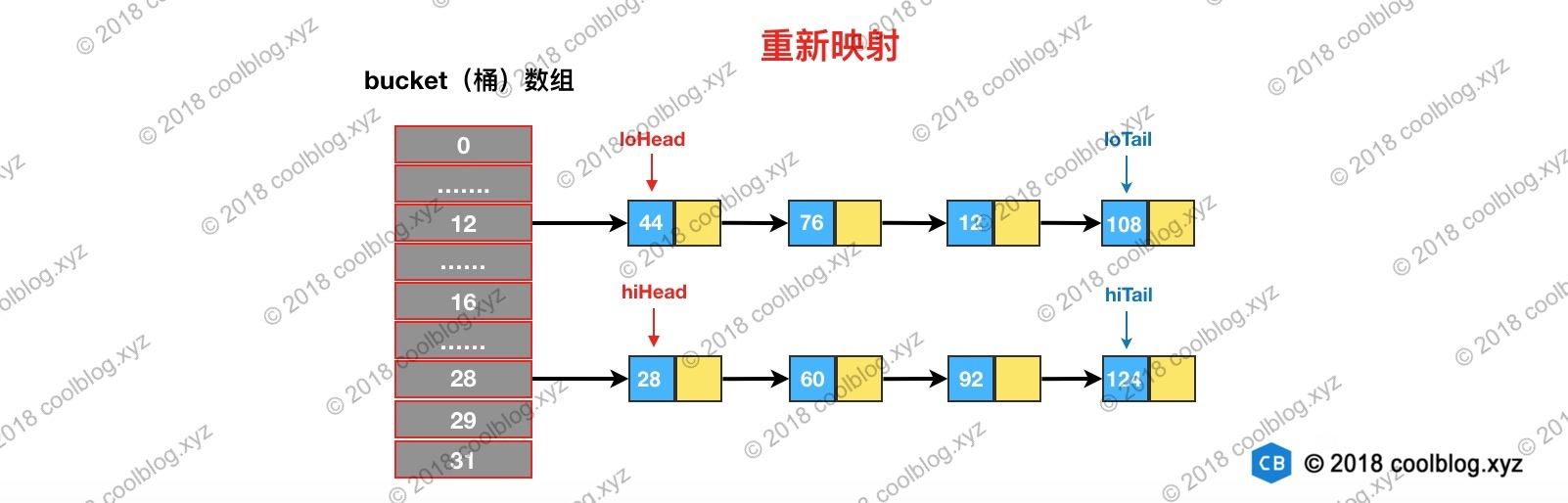##### 红黑树链化

final Node<K,V> untreeify(HashMap<K,V> map) {
Node<K,V> hd = null, tl = null;
// 遍历 TreeNode 链表，并用 Node 替换
for (Node<K,V> q = this; q != null; q = q.next) {
// 替换节点类型
Node<K,V> p = map.replacementNode(q, null);
if (tl == null)
hd = p;
else
tl.next = p;
tl = p;
}
return hd;
}

Node<K,V> replacementNode(Node<K,V> p, Node<K,V> next) {
return new Node<>(p.hash, p.key, p.value, next);
}

### 3.5 删除

HashMap 的删除操作并不复杂，仅需三个步骤即可完成。第一步是定位桶位置，第二步遍历链表并找到键值相等的节点，第三步删除节点。相关源码如下：

public V remove(Object key) {
Node<K,V> e;
return (e = removeNode(hash(key), key, null, false, true)) == null ?
null : e.value;
}

final Node<K,V> removeNode(int hash, Object key, Object value,
boolean matchValue, boolean movable) {
Node<K,V>[] tab; Node<K,V> p; int n, index;
if ((tab = table) != null && (n = tab.length) > 0 &&
// 1. 定位桶位置
(p = tab[index = (n - 1) & hash]) != null) {
Node<K,V> node = null, e; K k; V v;
// 如果键的值与链表第一个节点相等，则将 node 指向该节点
if (p.hash == hash &&
((k = p.key) == key || (key != null && key.equals(k))))
node = p;
else if ((e = p.next) != null) {
// 如果是 TreeNode 类型，调用红黑树的查找逻辑定位待删除节点
if (p instanceof TreeNode)
node = ((TreeNode<K,V>)p).getTreeNode(hash, key);
else {
// 2. 遍历链表，找到待删除节点
do {
if (e.hash == hash &&
((k = e.key) == key ||
(key != null && key.equals(k)))) {
node = e;
break;
}
p = e;
} while ((e = e.next) != null);
}
}

// 3. 删除节点，并修复链表或红黑树
if (node != null && (!matchValue || (v = node.value) == value ||
(value != null && value.equals(v)))) {
if (node instanceof TreeNode)
((TreeNode<K,V>)node).removeTreeNode(this, tab, movable);
else if (node == p)
tab[index] = node.next;
else
p.next = node.next;
++modCount;
--size;
afterNodeRemoval(node);
return node;
}
}
return null;
}

### 3.6 其他细节

#### 被 transient 所修饰 table 变量

1. table 多数情况下是无法被存满的，序列化未使用的部分，浪费空间
2. 同一个键值对在不同 JVM 下，所处的桶位置可能是不同的，在不同的 JVM 下反序列化 table 可能会发生错误。

## 参考JDK7 HashMap源码解析
JDK7 HashMap源码解析
25 051 0Jdk1.8 HashMap实现原理详细介绍
Jdk1.8 HashMap实现原理详细介绍
41 02022 最新 JDK 17 HashMap 源码解读 (一)
2022 最新 JDK 17 HashMap 源码解读 (一)
92 0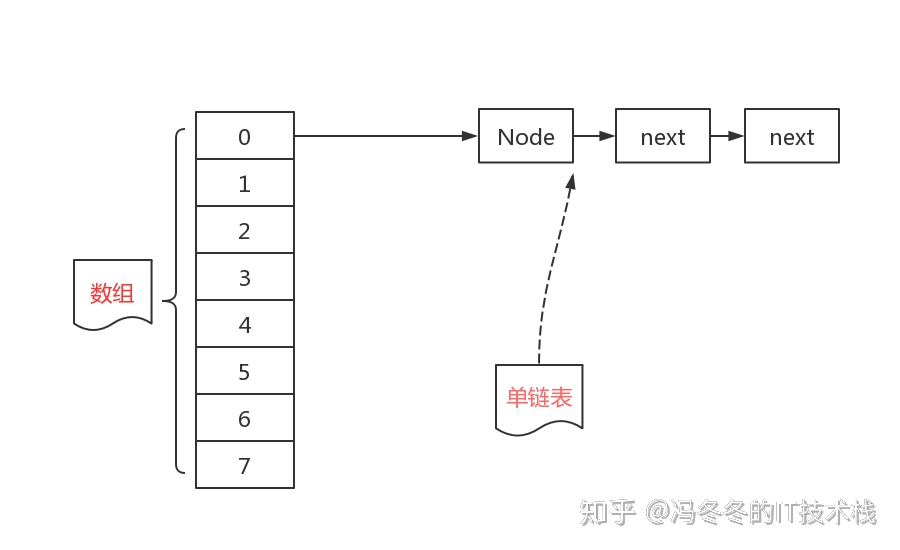HashMap源码分析（jdk1.8，保证你能看懂）

55 0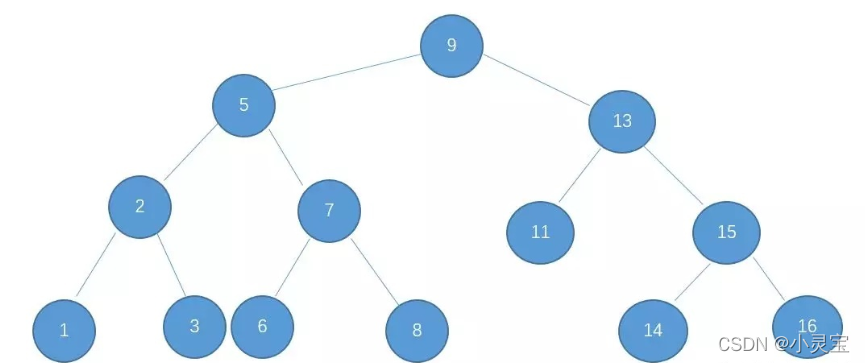HashMap源码解析（JDK1.8）
HashMap源码解析（JDK1.8）
37 0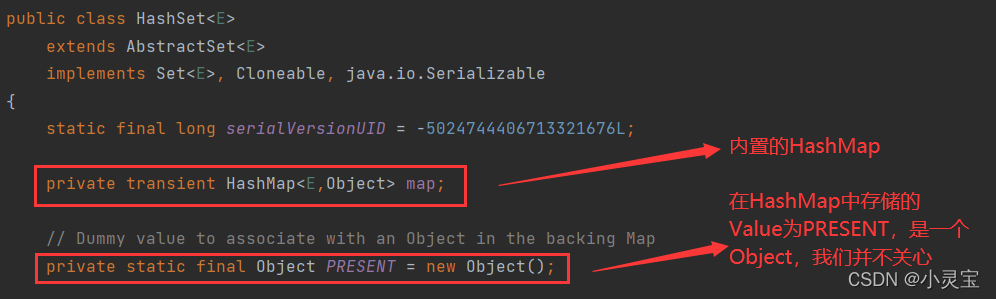HashSet源码解析（JDK1.8）
HashSet源码解析（JDK1.8）
39 0

JAVA反射原理以及一些常见的应用